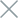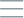• Home
• Tags
• Series
•# Local Search Algorithm in C++ - Explanation, Example and Code

The Local Search algorithm is a method for finding the best solution within a vicinity of the current state. This technique is often used to refine approximate solutions by iteratively modifying individual components to discover better states.

## How It Works

1. Initialization: Begin with an initial state.
2. Generate Neighbors: Generate neighboring states by changing a component of the current state.
3. Evaluation: Evaluate the quality of neighboring states using an objective function.
4. Select Best State: Choose the neighboring state with the best objective value.
5. Repeat: Iterate through steps 2 to 4 until no better neighboring state can be found.

## Example: Optimizing the Fibonacci Function

Consider the optimization problem of the Fibonacci function F(x) = F(x-1) + F(x-2) with F(0) = 0, F(1) = 1. We want to find the value of x for which F(x) is maximized. We can use the Local Search approach to iteratively explore farther from each step.

## Code Example in C++

``````#include <iostream>

int fibonacci(int n) {
if (n <= 0) return 0;
if (n == 1) return 1;
return fibonacci(n - 1) + fibonacci(n - 2);
}

int localSearchFibonacci(int maxIterations) {
int bestX = 0;
int bestValue = 0;

for (int x = 0; x < maxIterations; ++x) {
int value = fibonacci(x);
if (value > bestValue) {
bestValue = value;
bestX = x;
}
}

return bestX;
}

int main() {
int maxIterations = 20;
int result = localSearchFibonacci(maxIterations);

std::cout << "Optimal x for maximum Fibonacci value: " << result << std::endl;

return 0;
}
``````

In this example, we utilize the Local Search method to optimize the Fibonacci function. We iterate through different values of x and calculate the Fibonacci value at each x. When a better value is found, we update the best value and its corresponding x.